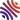## 35 publications1. Convergence acceleration of iterative sequences for equilibrium chemistry computations (, and ), In Computational Geosciences, .
2. Well posedness of general cross-diffusion systems (, and ), In Journal of Differential Equations, volume 300, . [hal]
3. Parameters Identification in a Saltwater Intrusion Problem ( and ), In Acta Mathematica Scientia, volume 40.
4. Modeling of shallow aquifers in interaction with overland water (, , and ), In Appl. Math. Model., volume 81, . [hal-lmpa]
5. A nonlinear optimization method applied to the hydraulic conductivity identification in unconfined aquifers ( and ), In J. Optim. Theory Appl., volume 183, .
6. A finite element method for a seawater intrusion problem in unconfined aquifers (, , and ), In Appl. Numer. Math., volume 127, .
7. Uniqueness for cross-diffusion systems issuing from seawater intrusion problems (, and ), In Electron. J. Differential Equations, . [hal-lmpa]
8. General decay rate estimates and numerical analysis for a transmission problem with locally distributed nonlinear damping (, and ), In Comput. Math. Appl., volume 73, .
9. Mathematical analysis of a seawater intrusion model including storativity (, and ), In SIAM J. Math. Anal., volume 49, . [hal]
10. Numerical analysis for the wave equation with locally nonlinear distributed damping (, and ), In J. Comput. Appl. Math., volume 301, .
11. Derivation of a sharp-diffuse interfaces model for seawater intrusion in a free aquifer. Numerical simulations (, and ), In SIAM J. Appl. Math., volume 76, . [hal-lmpa]
12. Numerical analysis for a seawater intrusion problem in a confined aquifer ( and ), In Math. Comput. Simulation, volume 118, .
13. Mathematical analysis of a sharp-diffuse interfaces model for seawater intrusion (, and ), In J. Differential Equations, volume 259, . [hal-lmpa]
14. Global existence for seawater intrusion models: comparison between sharp interface and sharp-diffuse interface approaches (, and ), In Electron. J. Differential Equations, .
15. Numerical simulations for a seawater intrusion problem in a free aquifer (, and ), In Appl. Numer. Math., volume 75, .
16. Effective models for reactive flow under a dominant Péclet number and order one Damköhler number: numerical simulations ( and ), In Nonlinear Anal. Real World Appl., volume 15, .
17. On the global existence for a degenerate elliptic-parabolic seawater intrusion problem ( and ), In Math. Comput. Simulation, volume 81, .
18. Smooth solutions for the motion of a ball in an incompressible perfect fluid ( and ), In J. Funct. Anal., volume 256, . [hal-lmpa]
19. Rigorous upscaling of the infinite adsorption rate reactive flow under dominant Peclet number through a pore ( and ), In Ann. Univ. Ferrara Sez. VII Sci. Mat., volume 53, .
20. On the global existence of solutions for a non-local problem occurring in statistical mechanics ( and ), In Nonlinear Anal., volume 60, . [hal-lmpa]
21. Modeling solute transport through unsaturated porous media using homogenization. I ( and ), In Comput. Appl. Math., volume 23, .
22. Global solutions for a problem modeling the dynamics of a system of self-gravitating Brownian particles ( and ), Chapter in Nonlocal elliptic and parabolic problems, Polish Acad. Sci. Inst. Math., Warsaw, volume 66, . [hal]
23. On the analogy between self-gravitating Brownian particles and bacterial populations (, , and ), Chapter in Nonlocal elliptic and parabolic problems, Polish Acad. Sci. Inst. Math., Warsaw, volume 66, . [hal]
24. Finite speed propagation in the relaxation of vortex patches ( and ), In Quart. Appl. Math., volume 61, .
25. Thermodynamics of self-gravitating systems (, and ), In Phys. Rev. E (3), volume 66, .
26. Problème de Cauchy pour une équation parabolique modélisant la relaxation des systèmes stellaires auto-gravitants (), In C. R. Acad. Sci. Paris Sér. I Math., volume 332, .
27. Well-posedness of a degenerate parabolic equation issuing from two-dimensional perfect fluid dynamics ( and ), In Appl. Anal., volume 75, .
28. The modeling of small scales in two-dimensional turbulent flows: a statistical mechanics approach ( and ), In J. Statist. Phys., volume 86, .
29. Multi-dimensional Riemann problems for linear hyperbolic systems (, and ), In RAIRO Modél. Math. Anal. Numér., volume 30, .
30. Spectral methods for $2$D Riemann problems ( and ), Chapter in , volume 116, .
31. Multi-dimensional Riemann problems for linear hyperbolic systems. II (, and ), Chapter in Nonlinear hyperbolic problems: theoretical, applied, and computational aspects (Taormina, 1992), Friedr. Vieweg, Braunschweig, volume 43, .
32. Multi-dimensional Riemann problems for linear hyperbolic systems. I (, and ), Chapter in Nonlinear hyperbolic problems: theoretical, applied, and computational aspects (Taormina, 1992), Friedr. Vieweg, Braunschweig, volume 43, .
33. A nonlinear Galerkin method for the Navier-Stokes equations (, and ), Chapter in Spectral and high order methods for partial differential equations (Como, 1989), North-Holland, Amsterdam, .
34. A nonlinear Galerkin method for the Navier-Stokes equations (, and ), Chapter in , volume 80, .
35. The nonlinear Galerkin method in computational fluid dynamics (, and ), In Appl. Numer. Math., volume 6, .